IMO Shortlist 2001 problem C2

Kvaliteta:
Avg: 0,0
Težina:
Avg: 6,0
Let$n$ be an odd integer greater than 1 and let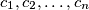$c_1, c_2, \ldots, c_n$ be integers. For each permutation$a = (a_1, a_2, \ldots, a_n)$ of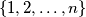$\{1,2,\ldots,n\}$, define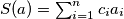$S(a) = \sum_{i=1}^n c_i a_i$. Prove that there exist permutations$a \neq b$ of$\{1,2,\ldots,n\}$ such that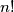$n!$ is a divisor of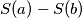$S(a)-S(b)$.
Izvor: Međunarodna matematička olimpijada, shortlist 2001